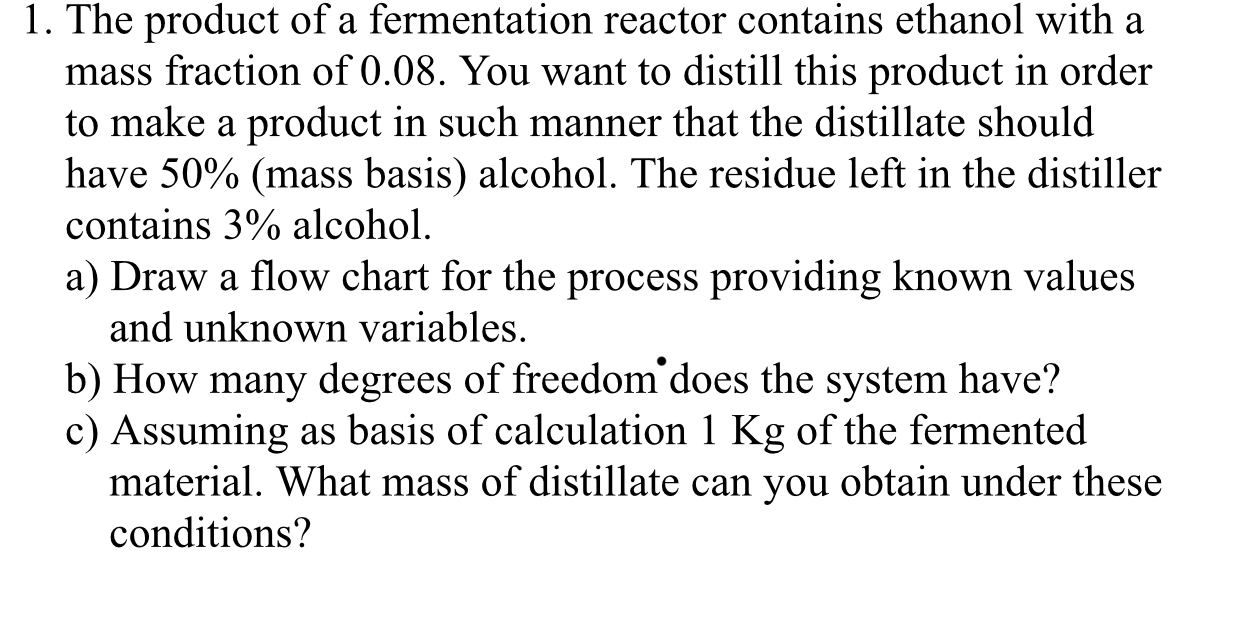1. The product of a fermentation reactor contains ethanol with amass fraction of 0.08. You want to distill this product in orderto make a product in such manner that the distillate shouldhave 50% (mass basis) alcohol. The residue left in the distillercontains 3% alcohola) Draw a flow chart for the process providing known valuesand unknown variables.b) How many degrees of freedom does the system have?c) Assuming as basis of calculation 1 Kg of the fermentedmaterial. What mass of distillate can you obtain under theseconditions?

Questionhelp_outlineImage Transcriptionclose1. The product of a fermentation reactor contains ethanol with a mass fraction of 0.08. You want to distill this product in order to make a product in such manner that the distillate should have 50% (mass basis) alcohol. The residue left in the distiller contains 3% alcohol a) Draw a flow chart for the process providing known values and unknown variables. b) How many degrees of freedom does the system have? c) Assuming as basis of calculation 1 Kg of the fermented material. What mass of distillate can you obtain under these conditions? fullscreen
Step 1

Part (a)

Let the mass of the fermenter products be mF kg which is fed to the distiller to produce mD kg of distillate and mR kg of residue.

The feed to the distiller contains 0.08 mass fraction of ethanol represented by ‘E’ and balance substance ‘A’.

In the distillate, ethanol present is 50% and in residue, it is 3%.

The flowchart representing this process is drawn below as:

Step 2

Part (b)

The formula used to calculate degree of freedom (DF) is written below.

Step 3

There are total 3 unknown variables present. These are, mF, mD, and mR.

There are 2 independent equations, one for each ...

Want to see the full answer?

See Solution

Want to see this answer and more?

Our solutions are written by experts, many with advanced degrees, and available 24/7

See Solution
Tagged in

Chemical Engineering